##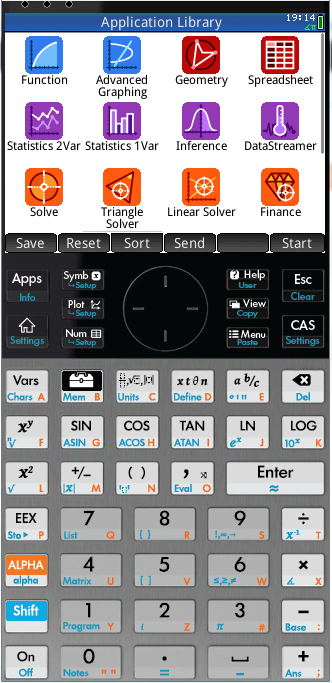HP Prime - Statistics 2Var App:  Manual and MAKE Data

 Introduction, HelpThe Statistics 2Var App is useful for performing regressions on sets of xy data both graphically and numerically  As usual, you should check out the online Help before using this feature.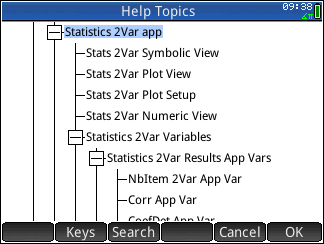In the examples below, we will generate and analyze 2 variable data by manually entering data and also by using the MAKE command.

## Example 1 - Manually enter x,y data - Chicago weather

If we open the Statistics 2Var App then click the Num view, we can enter numerical data directly as shown.  For this example, I just keyed into C1 ascending numbers 1-12 representing the month number.  For C2 and C3 I keyed in the average low and high monthly temperatures (deg C) in Chicago.

.Now that we have some data, we can move to the Symb to setup some 2 variable analysis.  If we want to plot C1 versus C2 and fit to a linear model, enter C1 and C2 into S1: as independent and dependant data as shown.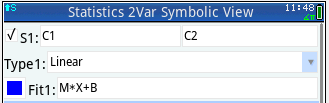Now press Symb and you should see a plot that looks “something” like this: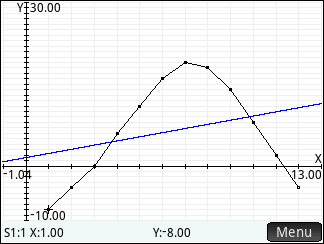It probably does not look like this unless you set up the Plot settings ahead of time.  To do this  Shift Plot (settings) will allow you to adjust the plot.  Here is what I used in the example for page 1 and 2: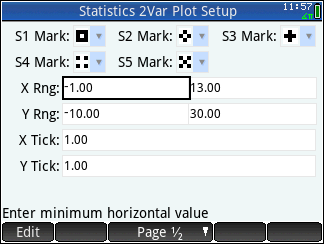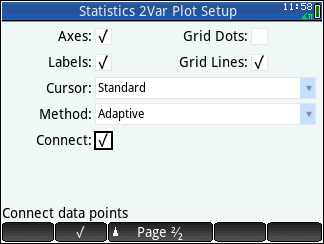If you want to see statistics related to the x, y and linear fit, pressing Plot Menu then Defn  can provide the linear model as shown Y = 0.76*X +1.55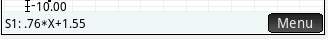Pressing  Num then stats will provide additional curve fit statistics.  In this case a linear fit is pretty poor.  Note that clicking on the X and Y will provide additional statistics.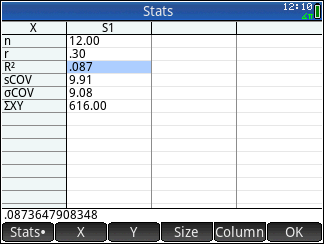Lets return to the Symb menu and see if we can analyze C2 versus C3 data (monthly low versus monthly high temperatures).  In this view, select C2 and C3 for S2:.  Make sure S2 is checked and S1 is now unchecked.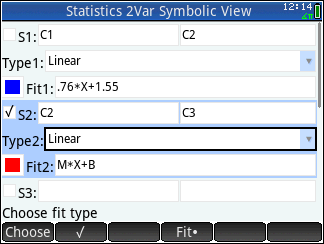Now press Plot and you will probably see something different that this -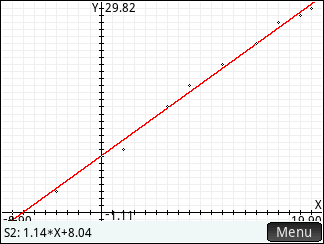After you pressed Plot , press Menu then Autoscale to get all the points in view.  Additionally, go to Shift Plot (settings) and uncheck Connect:,  Now it should look closer to plot above .  In this case, the model shows Y = 1.14*X +8.04 with what looks like a good fit.

As before, pressing  Num then stats will provide curve fit statistics.  In this case a linear fit is looks good.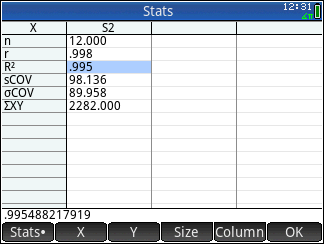## Example 2 - Use MAKE column data

In this example, we will generate another column of data into column 4 using Make column data within the Num view.  In the Num view, press the Make button which brings up a dialog box.  Here you can enter an expression that will generate data and store in a column for you.  Lets create data where the expression X0.5 + RANDNORM() is used and X goes from 1 to 12 in 1 unit steps.  After entering the expression and other information, the dialog box should look like this.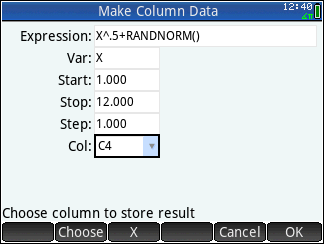Note that selecting RANDNORM() can be done by pressing Toolbox Math  5, 4, 3 as shown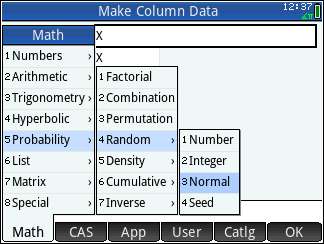Pressing OK generates new data in C4 as shown.  Yours may be different due random noise.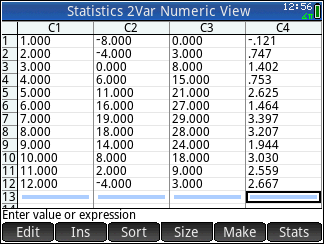Now, return to the Symb view and enter C1 and C4 for S3:   Uncheck S1,2: and check the S3: plot.  To get the plot below press Plot , Menu , Autoscale , then - key (zoom out) then dragged the plot to get it centered.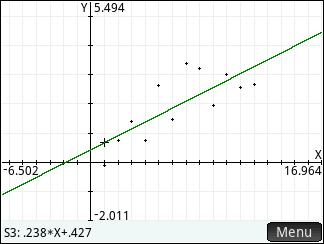This looks like a decent fit, but we may want to explore other fit models.

Try the following on your own:

• Try using different combinations of data sources for x, y data including manual C1 data, C2 data using MAKE, lists (L1, user variable) and lists generated by spreadsheets.
• Setup different spreadsheet apps for different data sets or experiments you want to keep a record of.  Perhaps SS DATA1, SS DATA2 etc.?
• In a spreadsheet, try generating y data (f(x) data) automatically based on x using spreadsheet formulas.  Can you add and control random noise?  See if the Statistics 2Var App can fit the model from the spreadsheet.

 You may have to hit the up or down arrow a couple of times to get to this equation.  You can also see the fit in the Symb view